Search Results (86)

View
Selected filters:
• Triangle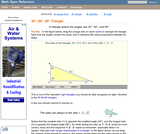Conditions of Use:
Read the Fine Print
Rating

An interactive applet and associated web page that demonstrate the properties of a 30-60-90 triangle. The applet shows a right triangle that can be resized by dragging any vertex. As it is dragged, the remaining vertices change so that the triangle's angles remain 30 degrees, 60 degrees and 90 degrees The text on the page points out that the sides of a 30-60-90 triangle are always in the ratio of 1 : 2 : root 3 Applet can be enlarged to full screen size for use with a classroom projector. This resource is a component of the Math Open Reference Interactive Geometry textbook project at http://www.mathopenref.com.

Subject:
Geometry
Material Type:
Simulation
Provider:
Math Open Reference
Author:
John Page
02/16/2011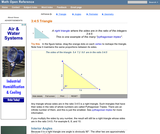Conditions of Use:
Read the Fine Print
Rating

An interactive applet and associated web page that demonstrate the properties of a 3:4:5 triangle - one of the Pythagorean triples. The applet shows a right triangle that can be resized by dragging any vertex. As it is dragged, the remaining vertices change so that the triangle's side remain in the ration 3:4:5. The text on the page has an example of how the triangle can be used to measure a right angle on even large objects. Applet can be enlarged to full screen size for use with a classroom projector. This resource is a component of the Math Open Reference Interactive Geometry textbook project at http://www.mathopenref.com.

Subject:
Geometry
Material Type:
Simulation
Provider:
Math Open Reference
Author:
John Page
02/16/2011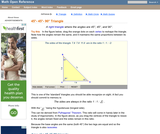Conditions of Use:
Read the Fine Print
Rating

An interactive applet and associated web page that demonstrate the properties of a 45-45-90 isosceles right triangle. The applet shows a right triangle that can be resized by dragging any vertex. As it is dragged, the remaining vertices change so that the triangle's angles remain 45 degrees, 45 degrees and 90 degrees The text on the page points out that the sides of a 45-45-90 triangle are always in the ratio of 1 : 2 : root 2 Applet can be enlarged to full screen size for use with a classroom projector. This resource is a component of the Math Open Reference Interactive Geometry textbook project at http://www.mathopenref.com.

Subject:
Geometry
Material Type:
Simulation
Provider:
Math Open Reference
Author:
John Page
02/16/2011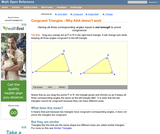Conditions of Use:
Read the Fine Print
Rating

An interactive applet and associated web page that shows that angle-angle-angle (AAA) is not enough to prove congruence. The applet shows two triangles, one of which can be dragged to resize it, showing that although they have the same angles they are not the same size and thus not congruent. The web page describes all this and has links to other related pages. Applet can be enlarged to full screen size for use with a classroom projector. This resource is a component of the Math Open Reference Interactive Geometry textbook project at http://www.mathopenref.com.

Subject:
Geometry
Material Type:
Simulation
Provider:
Math Open Reference
Author:
John Page
02/16/2011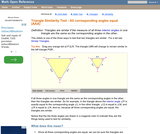Conditions of Use:
Read the Fine Print
Rating

An interactive applet and associated web page showing how the AAA similarity test works. Two similar triangles are shown that can be resized by dragging. The other triangle adjusts to remain similar and the angle-angle-angle elements are highlighted to show how they are involved in this test of similarity. (all three interior angles congruent). The web page describes all this and has links to other related pages. Applet can be enlarged to full screen size for use with a classroom projector. This resource is a component of the Math Open Reference interactive geometry reference book project at http://www.mathopenref.com.

Subject:
Geometry
Material Type:
Simulation
Provider:
Math Open Reference
Author:
John Page
02/16/2011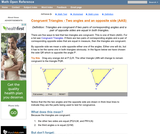Conditions of Use:
Read the Fine Print
Rating

An interactive applet and associated web page that shows how triangles that have two angles and a non-included side the same must be congruent. The applet shows two triangles, one of which can be reshaped by dragging any vertex. The other changes to remain congruent to it and the two angles and non-included side are outlined in bold to show they are the same measure and are the elements being used to prove congruence. The web page describes all this and has links to other related pages. Applet can be enlarged to full screen size for use with a classroom projector. This resource is a component of the Math Open Reference Interactive Geometry textbook project at http://www.mathopenref.com.

Subject:
Geometry
Material Type:
Simulation
Provider:
Math Open Reference
Author:
John Page
02/16/2011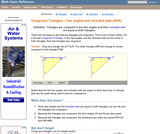Conditions of Use:
Read the Fine Print
Rating

An interactive applet and associated web page that shows how triangles that have two angles and their included side the same must be congruent. The applet shows two triangles, one of which can be reshaped by dragging any vertex. The other changes to remain congruent to it and the two angles and the included side are outlined in bold to show they are the same measure and are the elements being used to prove congruence. The web page describes all this and has links to other related pages. Applet can be enlarged to full screen size for use with a classroom projector. This resource is a component of the Math Open Reference Interactive Geometry textbook project at http://www.mathopenref.com.

Subject:
Geometry
Material Type:
Simulation
Provider:
Math Open Reference
Author:
John Page
02/16/2011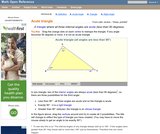Conditions of Use:
Read the Fine Print
Rating

An interactive applet and associated web page that demonstrate the three types of triangle: acute, obtuse and right. The applet shows a triangle that is initially acute (all angles less then 90 degrees) which the user can reshape by dragging any vertex. There is a message changes in real time while the triangle is being dragged that tells if the triangle is an acute, right or obtuse triangle and gives the reason why. By experimenting with the triangle student can develop an intuitive sense of the difference between these three classes of triangle. Applet can be enlarged to full screen size for use with a classroom projector. This resource is a component of the Math Open Reference Interactive Geometry textbook project at http://www.mathopenref.com.

Subject:
Geometry
Material Type:
Simulation
Provider:
Math Open Reference
Author:
John Page
02/16/2011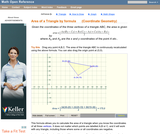Conditions of Use:
Read the Fine Print
Rating

An interactive applet and associated web page that calculate the area of a triangle using the formula method in coordinate geometry. The applet has a triangle with draggable vertices. As you drag them the triangle's area is recalculated from the vertex coordinates using the formula. The grid and coordinates can be turned on and off. The area calculation can be turned off to permit class exercises and then turned back on the verify the answers. The applet can be printed as it appears on the screen to make handouts. The web page has a full description of the method for determining area using the formula method, a worked example and has links to other pages relating to coordinate geometry. Applet can be enlarged to full screen size for use with a classroom projector. This resource is a component of the Math Open Reference Interactive Geometry textbook project at http://www.mathopenref.com.

Subject:
Geometry
Material Type:
Simulation
Provider:
Math Open Reference
Author:
John Page
02/16/2011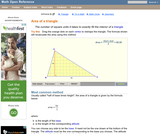Conditions of Use:
Read the Fine Print
Rating

An interactive applet and associated web page that explain the area of a triangle. The applet shows a triangle that can be reshaped by dragging any vertex. As it changes, the area is continually recalculated using the 'half base times height' method. The triangle has a fixed square grid in its interior that can be used to visually estimate the area for later correlation with the calculated value. The calculation can be hidden while estimation is in progress. The text page has links to a similar page that uses Heron's Formula to compute the area. Applet can be enlarged to full screen size for use with a classroom projector. This resource is a component of the Math Open Reference Interactive Geometry textbook project at http://www.mathopenref.com.

Subject:
Geometry
Material Type:
Simulation
Provider:
Math Open Reference
Author:
John Page
02/16/2011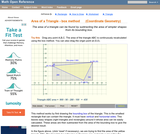Conditions of Use:
Read the Fine Print
Rating

An interactive applet and associated web page that calculate the area of a triangle using the box method in coordinate geometry. The applet has a triangle with draggable vertices. As you drag them the triangle's bounding box is shown and the area recalculated by subtracting the areas of the outside triangles. The grid and coordinates can be turned on and off. The area calculation can be turned off to permit class exercises and then turned back on the verify the answers. The applet can be printed as it appears on the screen to make handouts. The web page has a full description of the method for determining area using the box method, a worked example and has links to other pages relating to coordinate geometry. Applet can be enlarged to full screen size for use with a classroom projector. This resource is a component of the Math Open Reference Interactive Geometry textbook project at http://www.mathopenref.com.

Subject:
Geometry
Material Type:
Simulation
Provider:
Math Open Reference
Author:
John Page
02/16/2011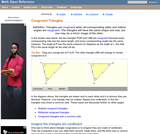Conditions of Use:
Read the Fine Print
Rating

An interactive applet and associated web page that demonstrate the concept of congruent triangles. Applets show that triangles a re congruent if the are the same, rotated, or reflected. In each case the user can drag one triangle and see how another triangle changes to remain congruent to it. The web page describes all this and has links to other related pages. Applet can be enlarged to full screen size for use with a classroom projector. This resource is a component of the Math Open Reference Interactive Geometry textbook project at http://www.mathopenref.com.

Subject:
Geometry
Material Type:
Simulation
Provider:
Math Open Reference
Author:
John Page
02/16/2011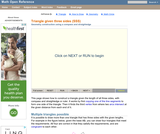Conditions of Use:
Read the Fine Print
Rating

An interactive applet and associated web page that provide step-by-step animated instructions on how to construct a triangle given all three sides (SSS), using only a compass and straightedge. The animation can be run either continuously like a video, or single stepped to allow classroom discussion and thought between steps. Applet can be enlarged to full screen size for use with a classroom projector. This resource is a component of the Math Open Reference Interactive Geometry textbook project at http://www.mathopenref.com.

Subject:
Geometry
Material Type:
Simulation
Provider:
Math Open Reference
Author:
John Page
02/16/2011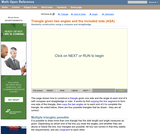Conditions of Use:
Read the Fine Print
Rating

An interactive applet and associated web page that provide step-by-step animated instructions on how to construct a triangle given two angles and the included side length (ASA), using only a compass and straightedge. The animation can be run either continuously like a video, or single stepped to allow classroom discussion and thought between steps. Applet can be enlarged to full screen size for use with a classroom projector. This resource is a component of the Math Open Reference Interactive Geometry textbook project at http://www.mathopenref.com.

Subject:
Geometry
Material Type:
Simulation
Provider:
Math Open Reference
Author:
John Page
02/16/2011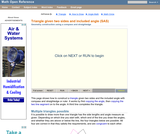Conditions of Use:
Read the Fine Print
Rating

An interactive applet and associated web page that provide step-by-step animated instructions on how to construct a triangle given two sides and the included angle (SAS), using only a compass and straightedge. The animation can be run either continuously like a video, or single stepped to allow classroom discussion and thought between steps. Applet can be enlarged to full screen size for use with a classroom projector. This resource is a component of the Math Open Reference Interactive Geometry textbook project at http://www.mathopenref.com.

Subject:
Geometry
Material Type:
Simulation
Provider:
Math Open Reference
Author:
John Page
02/16/2011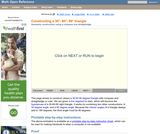Conditions of Use:
Read the Fine Print
Rating

An interactive applet and associated web page that provide step-by-step animated instructions on how to construct 30-60-90 triangle with given base length using only a compass and straightedge. The animation can be run either continuously like a video, or single stepped to allow classroom discussion and thought between steps. Applet can be enlarged to full screen size for use with a classroom projector. This resource is a component of the Math Open Reference Interactive Geometry textbook project at http://www.mathopenref.com.

Subject:
Geometry
Material Type:
Simulation
Provider:
Math Open Reference
Author:
John Page
02/16/2011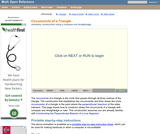Conditions of Use:
Read the Fine Print
Rating

An interactive applet and associated web page that provide step-by-step instructions on how to find the circumcenter and circumcircle of a triangle using only a compass and straightedge. The method used involves constructing the perpendicular bisectors of two of the triangle's sides. The bisectors intersect at the circumcenter of the triangle. The animation can be run either continuously like a video, or single stepped to allow classroom discussion and thought between steps. Applet can be enlarged to full screen size for use with a classroom projector. This resource is a component of the Math Open Reference Interactive Geometry textbook project at http://www.mathopenref.com.

Subject:
Geometry
Material Type:
Simulation
Provider:
Math Open Reference
Author:
John Page
02/16/2011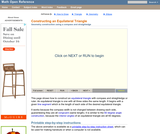Conditions of Use:
Read the Fine Print
Rating

An interactive applet and associated web page that provide step-by-step instructions on how to construct an equilateral triangle with a given side length using only a compass and straightedge. The animation can be run either continuously like a video, or single stepped to allow classroom discussion and thought between steps. Applet can be enlarged to full screen size for use with a classroom projector. This resource is a component of the Math Open Reference Interactive Geometry textbook project at http://www.mathopenref.com.

Subject:
Geometry
Material Type:
Simulation
Provider:
Math Open Reference
Author:
John Page
02/16/2011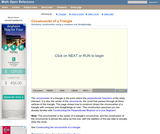Conditions of Use:
Read the Fine Print
Rating

An interactive applet and associated web page that provide step-by-step animated instructions on how to construct the circumcenter of a triangle. The animation can be run either continuously like a video, or single stepped to allow classroom discussion and thought between steps. Applet can be enlarged to full screen size for use with a classroom projector. This resource is a component of the Math Open Reference Interactive Geometry textbook project at http://www.mathopenref.com.

Subject:
Geometry
Material Type:
Simulation
Provider:
Math Open Reference
Author:
John Page
02/16/2011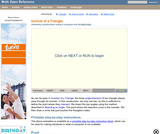Conditions of Use:
Read the Fine Print
Rating

An interactive applet and associated web page that demonstrate how to construct the incenter and incircle of triangle using compass and straightedge. It starts with a given triangle, and using animation that can be run continuously or single step, shows how to first construct the incenter, then using that point, construct the incircle of the given triangle. The text on the page contains written step-by-step instructions that can be printed. Applet can be enlarged to full screen size for use with a classroom projector. This resource is a component of the Math Open Reference Interactive Geometry textbook project at http://www.mathopenref.com.

Subject:
Geometry
Material Type:
Simulation
Provider:
Math Open Reference
Author:
John Page
02/16/2011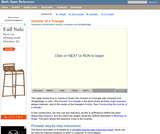Conditions of Use:
Read the Fine Print
Rating

An interactive applet and associated web page that provide step-by-step animated instructions on how to construct the incenter of a triangle. The animation can be run either continuously like a video, or single stepped to allow classroom discussion and thought between steps. Applet can be enlarged to full screen size for use with a classroom projector. This resource is a component of the Math Open Reference Interactive Geometry textbook project at http://www.mathopenref.com.

Subject:
Geometry
Material Type:
Simulation
Provider:
Math Open Reference
Author:
John Page
02/16/2011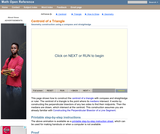Conditions of Use:
Read the Fine Print
Rating

An interactive applet and associated web page that provide step-by-step instructions on how to find the centroid of a triangle using only a compass and straightedge. The method used involves constructing two of the triangle's medians. The centroid is where they intersect. The animation can be run either continuously like a video, or single stepped to allow classroom discussion and thought between steps. Applet can be enlarged to full screen size for use with a classroom projector. This resource is a component of the Math Open Reference Interactive Geometry textbook project at http://www.mathopenref.com.

Subject:
Geometry
Material Type:
Simulation
Provider:
Math Open Reference
Author:
John Page
02/16/2011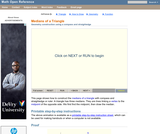Conditions of Use:
Read the Fine Print
Rating

An interactive applet and associated web page that provide step-by-step instructions on how to find a median of a triangle using only a compass and straightedge. The method used involves bisecting one side, then drawing a line from this midpoint to the opposite vertex. The animation can be run either continuously like a video, or single stepped to allow classroom discussion and thought between steps. Applet can be enlarged to full screen size for use with a classroom projector. This resource is a component of the Math Open Reference Interactive Geometry textbook project at http://www.mathopenref.com.

Subject:
Geometry
Material Type:
Simulation
Provider:
Math Open Reference
Author:
John Page
02/16/2011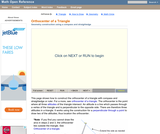Conditions of Use:
Read the Fine Print
Rating

An interactive applet and associated web page that provide step-by-step instructions on how to construct the orthocenter of a triangle using only a compass and straightedge. The method used involves constructing two altitudes of the triangle, which intersect at the orthocenter. The animation can be run either continuously like a video, or single stepped to allow classroom discussion and thought between steps. Applet can be enlarged to full screen size for use with a classroom projector. This resource is a component of the Math Open Reference Interactive Geometry textbook project at http://www.mathopenref.com.

Subject:
Geometry
Material Type:
Simulation
Provider:
Math Open Reference
Author:
John Page
02/16/2011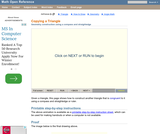Conditions of Use:
Read the Fine Print
Rating

An interactive applet and associated web page that provide step-by-step instructions on how to copy a given triangle using only a compass and straightedge. The animation can be run either continuously like a video, or single stepped to allow classroom discussion and thought between steps. Applet can be enlarged to full screen size for use with a classroom projector. This resource is a component of the Math Open Reference Interactive Geometry textbook project at http://www.mathopenref.com.

Subject:
Geometry
Material Type:
Simulation
Provider:
Math Open Reference
Author:
John Page
02/16/2011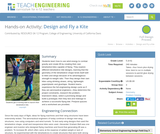Conditions of Use:
Read the Fine Print
Rating

Students learn how to use wind energy to combat gravity and create lift by creating their own tetrahedral kites capable of flying. They explore different tetrahedron kite designs, learning that the geometry of the tetrahedron shape lends itself well to kites and wings because of its advantageous strength-to-weight ratio. Then they design their own kites using drinking straws, string, lightweight paper/plastic and glue/tape. Student teams experience the full engineering design cycle as if they are aeronautical engineers—they determine the project constraints, research the problem, brainstorm ideas, select a promising design and build a prototype; then they test and redesign to achieve a successful flying kite. Pre/post quizzes and a worksheet are provided.

Subject:
Mathematics
Geometry
Material Type:
Activity/Lab
Provider:
TeachEngineering
Provider Set:
TeachEngineering
Author:
Joshua T. Claypool
02/17/2017Conditions of Use:
Read the Fine Print
Rating

Students use simple materials to design an open spectrograph so they can calculate the angle light is bent when it passes through a holographic diffraction grating. A holographic diffraction grating acts like a prism, showing the visual components of light. After finding the desired angles, students use what they have learned to design their own spectrograph enclosure.

Subject:
Engineering
Physics
Material Type:
Activity/Lab
Provider:
TeachEngineering
Provider Set:
TeachEngineering
10/14/2015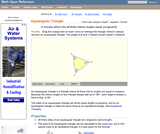Conditions of Use:
Read the Fine Print
Rating

An interactive applet and associated web page that demonstrate equiangular triangles (all interior angles congruent). The applet presents an equiangular triangle where the user can drag any vertex. As the vertex is dragged, the others move automatically to keep the triangle equiangular. The angles and side lengths are updated continuously to show that the all interior angles are always congruent. Applet can be enlarged to full screen size for use with a classroom projector. This resource is a component of the Math Open Reference Interactive Geometry textbook project at http://www.mathopenref.com.

Subject:
Geometry
Material Type:
Simulation
Provider:
Math Open Reference
Author:
John Page
02/16/2011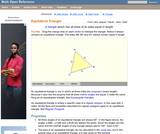Conditions of Use:
Read the Fine Print
Rating

An interactive applet and associated web page that demonstrate equilateral triangles (all sides the same length). The applet presents an equilateral triangle where the user can drag any vertex. As the vertex is dragged, the others move automatically to keep the triangle equilateral. The angles are also updated continuously to show that the all interior angles are always congruent. Applet can be enlarged to full screen size for use with a classroom projector. This resource is a component of the Math Open Reference Interactive Geometry textbook project at http://www.mathopenref.com.

Subject:
Geometry
Material Type:
Simulation
Provider:
Math Open Reference
Author:
John Page
02/16/2011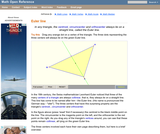Conditions of Use:
Read the Fine Print
Rating

An interactive applet and associated web page that describe the Euler line. It shows a triangle whose vertices can be dragged. As you drag them, the centroid, circumcenter and orthocenter move appropriately, showing that they are always collinear, always lying on the Euler line. There are controls to individually turn on the construction lines for each center. This helps visualize the concept since if they are all drawn at once, the diagram would be hopelessly confused. The web page has a full description the Euler line and has links to separate pages which go into each center in depth. Applet can be enlarged to full screen size for use with a classroom projector. Diagram can be printed. This resource is a component of the Math Open Reference Interactive Geometry textbook project at http://www.mathopenref.com.

Subject:
Geometry
Material Type:
Simulation
Provider:
Math Open Reference
Author:
John Page
02/16/2011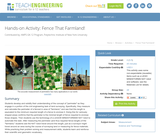Conditions of Use:
Read the Fine Print
Rating

Students develop and solidify their understanding of the concept of "perimeter" as they engage in a portion of the civil engineering task of land surveying. Specifically, they measure and calculate the perimeter of a fenced in area of "farmland," and see that this length is equivalent to the minimum required length of a fence to enclose it. Doing this for variously shaped areas confirms that the perimeter is the minimal length of fence required to enclose those shapes. Then students use the technology of a LEGO MINDSTORMS(TM) NXT robot to automate this task. After measuring the perimeter (and thus required fence length) of the "farmland," students see the NXT robot travel around this length, just as a surveyor might travel around an area during the course of surveying land or measuring for fence materials. While practicing their problem solving and measurement skills, students learn and reinforce their scientific and geometric vocabulary.

Subject:
Engineering
Material Type:
Activity/Lab
Provider:
TeachEngineering
Provider Set:
TeachEngineering
Author:
Ursula Koniges
09/18/2014Conditions of Use:
Remix and Share
Rating

Origami is the Japanese art of paper folding, originally from China and now practiced all over the world. In this activity, create an origami star. Introduce children to both geometry and analytical skills in a creative way.

Subject:
Arts and Humanities
Astronomy
Material Type:
Activity/Lab
Game
Provider:
UNAWE
Provider Set:
EU Universe Awareness
03/20/2012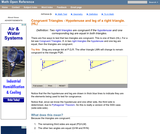Conditions of Use:
Read the Fine Print
Rating

An interactive applet and associated web page that shows how right triangles that have the hypotenuse and one other leg the same length must be congruent. The applet shows two right triangles, one of which can be reshaped by dragging any vertex. The other changes to remain congruent to it, and the hypotenuse and leg are outlined in bold to show they are the same measure and are the elements being used to prove congruence. The web page describes all this and has links to other related pages. Applet can be enlarged to full screen size for use with a classroom projector. This resource is a component of the Math Open Reference Interactive Geometry textbook project at http://www.mathopenref.com.

Subject:
Geometry
Material Type:
Simulation
Provider:
Math Open Reference
Author:
John Page
02/16/2011Conditions of Use:
Remix and Share
Rating

In this activity, learners use their hands as tools for indirect measurement. Learners explore how to use ratios to calculate the approximate height of something that can't be measured directly by first measuring something that can be directly measured. This activity can also be used to explain how scientists use indirect measurement to determine distances between things in the universe that are too far away, too large or too small to measure directly (i.e. diameter of the moon or number of bacteria in a volume of liquid).

Subject:
Mathematics
Material Type:
Activity/Lab
Provider:
Exploratorium
Author:
Exploratorium
Gordon and Betty Moore Foundation
National Science Foundation
The Exploratorium
12/07/2010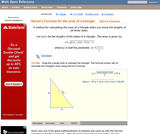Conditions of Use:
Read the Fine Print
Rating

An interactive applet and associated web page that demonstrate how Herons Formula can be used to find the area of a triangle when you know all three sides. A triangle is shown where the user can drag any vertex to reshape it. In real time while dragging, the side lengths change and the formula is recalculated on the screen. The formula can be turned off to facilitate class work. The text on the web page gives the full formula and discusses where is can be applied. Applet can be enlarged to full screen size for use with a classroom projector. This resource is a component of the Math Open Reference Interactive Geometry textbook project at http://www.mathopenref.com.

Subject:
Geometry
Material Type:
Simulation
Provider:
Math Open Reference
Author:
John Page
02/16/2011Rating

Subject:
Geometry
Material Type:
Lesson Plan
Author:
summer dickerhoof
12/07/2017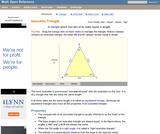Conditions of Use:
Read the Fine Print
Rating

An interactive applet and associated web page that demonstrate isosceles triangles (two sides the same length). The applet presents a triangle where the user can drag any vertex. As the vertex is dragged the others move automatically to keep the triangle isosceles. The angles are also updated continuously to show that the base angles are always congruent. Applet can be enlarged to full screen size for use with a classroom projector. This resource is a component of the Math Open Reference Interactive Geometry textbook project at http://www.mathopenref.com.

Subject:
Geometry
Material Type:
Simulation
Provider:
Math Open Reference
Author:
John Page
02/16/2011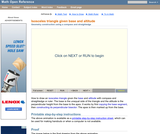Conditions of Use:
Read the Fine Print
Rating

An interactive applet and associated web page that provide step-by-step animated instructions on how to construct an isosceles triangle with given base and altitude using only a compass and straightedge. The animation can be run either continuously like a video, or single stepped to allow classroom discussion and thought between steps. Applet can be enlarged to full screen size for use with a classroom projector. This resource is a component of the Math Open Reference Interactive Geometry textbook project at http://www.mathopenref.com.

Subject:
Geometry
Material Type:
Simulation
Provider:
Math Open Reference
Author:
John Page
02/16/2011Conditions of Use:
Read the Fine Print
Rating

An interactive applet and associated web page that provide step-by-step animated instructions on how to construct an isosceles triangle with given sides using only a compass and straightedge. The animation can be run either continuously like a video, or single stepped to allow classroom discussion and thought between steps. Applet can be enlarged to full screen size for use with a classroom projector. This resource is a component of the Math Open Reference Interactive Geometry textbook project at http://www.mathopenref.com.

Subject:
Geometry
Material Type:
Simulation
Provider:
Math Open Reference
Author:
John Page
02/16/2011Conditions of Use:
Read the Fine Print
Rating

Students learn about catapults, including the science and math concepts behind them, as they prepare for the associated activity in which they design, build and test their own catapults. They learn about force, accuracy, precision and angles.

Subject:
Engineering
Physics
Material Type:
Lesson Plan
Provider:
TeachEngineering
Provider Set:
TeachEngineering
Author:
Carleigh Samson
Jake Crosby
Jonathan McNeil
Malinda Schaefer Zarske
William Surles